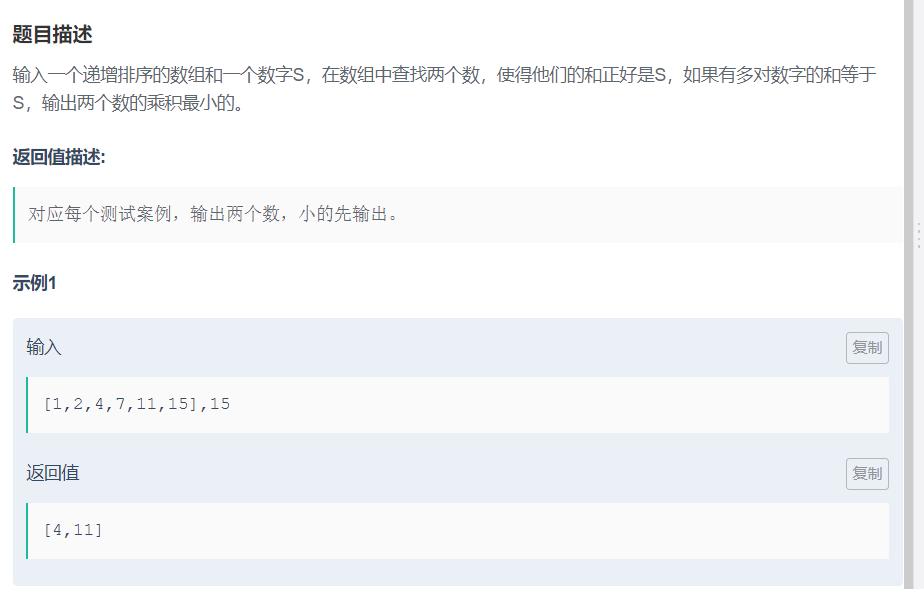剑指offer之和为S的两个数字public ArrayList<Integer> FindNumbersWithSum(int [] array, int sum) {

int i = 0;
int j = array.length-1;
ArrayList<Integer> list = new ArrayList<>();
while (i<=j) {

if (array[i]+array[j]<sum) {

i++;
continue;
}
while (array[i]+array[j]>sum) {

j--;
}
if (array[i]+array[j] == sum) {

return list;
}
i++;
}
return list;

}